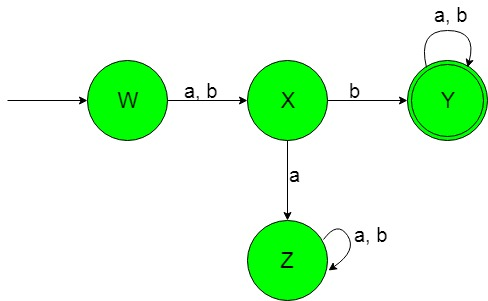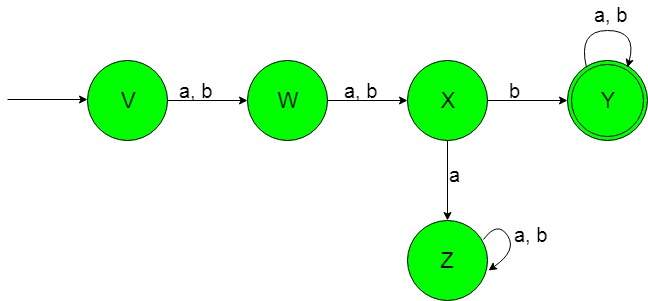# Designing Deterministic Finite Automata (Set 8)

• Last Updated : 20 Nov, 2019

Prerequisite: Designing finite automata
In this article, we will see some designing of Deterministic Finite Automata (DFA).

Problem-1: Construction of a minimal DFA accepting a set of strings over {a, b} in which the second symbol from left-hand side is always ‘b’.
Explanation: The desired language will be like:

Attention reader! Don’t stop learning now.  Practice GATE exam well before the actual exam with the subject-wise and overall quizzes available in GATE Test Series Course.

Learn all GATE CS concepts with Free Live Classes on our youtube channel.

`L1 = {ab, aba, abaa, bb, bb, bbbb, ...........}`

Here as we can see that each string of the language containing ‘b’ as the second symbol from left-hand side but the below language is not accepted by this DFA because some of the string of the below language does not contain ‘b’ as the second symbol from the left-hand side.

`L2 = {ba, ba, babaaa..............}`

This language L2 is not accepted by this required DFA because it does not contain ‘b’ as the second symbol from the left-hand side.
The state transition diagram of the desired language will be like below:In the above DFA, The state ‘W’ is the initial state which on getting either ‘a’ or ‘b’ as the input it transit to a state ‘X’. The state ‘X’ on getting ‘b’ as the input it transits to the final state ‘Y’ and on getting ‘a’ as the input it transit to a dead state ‘Z’.

The final state ‘Y’ on getting either ‘a’ or ‘b’ as the input it remains in the state of itself. The dead state ‘Z’ is called dead because it can not go to the final state on getting any of the input alphabets.

Note: The number of states in the above DFA is (n+2), where ‘n’ is the number from the left-hand side of the string used in the above language.

Problem-2: Construction of a minimal DFA accepting a set of strings over {a, b} in which the third symbol from left-hand side is always ‘b’.
Explanation: The desired language will be like:

`L1 = {aab, baba, aabaa, bbb, abb, bbbb, ...........}`

Here as we can see that each string of the language containing ‘b’ as the third symbol from left-hand side but the below language is not accepted by this DFA because some of the string of the below language does not contain ‘b’ as the third symbol from the left-hand side.

`L2 = {baa, aba, baabaaa..............}`

This language L2 is not accepted by this required DFA because it does not contain ‘b’ as the third symbol from the left-hand side.
The state transition diagram of the desired language will be like below:In the above DFA, The state ‘V’ is the initial state which on getting either ‘a’ or ‘b’ as the input it transits to the state ‘W’. The state ‘W’ is a state which on getting either ‘a’ or ‘b’ as the input it transits to a state ‘X’. The state ‘X’ on getting ‘b’ as the input it transits to the final state ‘Y’ and on getting ‘a’ as the input it transit to a dead state ‘Z’.

The final state ‘Y’ on getting either ‘a’ or ‘b’ as the input it remains in the state of itself. The dead state ‘Z’ is called dead because it can not go to the final state on getting any of the input alphabets.

Note: The number of states in the above DFA is (n+2), where ‘n’ is the number from the left-hand side of the string used in the above language.

My Personal Notes arrow_drop_up